Gaurav Singhal

# Encoders and Decoders for Neural Machine Translation

• Nov 19, 2020
• 3,474 Views
• Nov 19, 2020
• 3,474 Views

## Introduction

There are over 7,000 languages in the world. However, only 23 languages in total are most spoken around the globe, including English, Mandarin Chinese, Hindi, and Spanish. As the world is connecting faster, language translation bridges the communication gap.Google Translate can translate not only text but also speech and images in real-time. You can use it on your laptop, mobile, or even smartwatch. This guide will show the technology behind this magic.

Before moving further, I would recommend reading these guides on RNN and LSTM.

To follow along with this guide, download and unzip the `spa-eng.zip` file here. You will only use the spa.txt file for this process.

Let's get started.

## The Power of Sequence2Sequence (seq2seq) Modeling

There are multiple tasks that can be solved by using seq2seq modeling, including text summarization, speech recognition, image and video captioning, and question answering. It can also be used in genomics for DNA sequence modeling. A seq2seq model has two parts: an encoder and a decoder. Both work separately and come together to form a huge neural network model.

This architecture has the ability to handle the input and output sequences of variable length. The below image shows the types of RNN models and their use cases.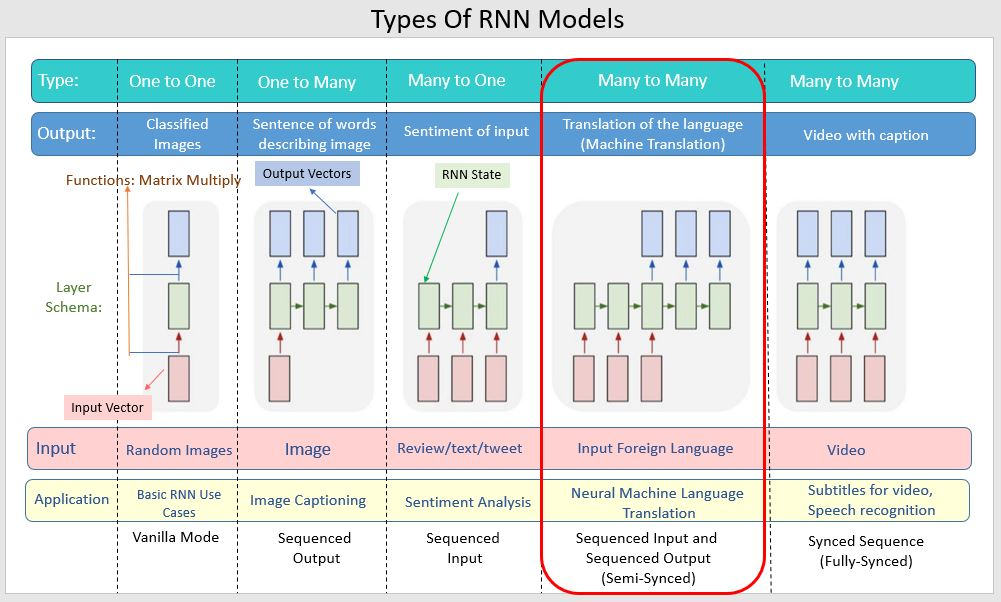## Encoder and Decoder

The following sections will cover encoder-decoder in-depth.

### Encoder

The encoder is at the feeding end; it understands the sequence and reduces the dimension of the input sequence. The sequence has a fixed size known as the context vector. This context vector acts like input to the decoder, which generates an output sequence when reaching the end token. Hence, you can call these seq2seq models encoder-decoder models.

This architecture can handle input and output sequences of variable length.

### Decoder

If you use LSTM for the encoder, use the same for the decoder. But it's slightly more complex than the encoder network. You can say the decoder is in an "aware state." It knows what words you have generated so far and what the previous hidden state was. The first layer of the decoder is initialized by using the context vector 'C' from the encoder network to generate the output. Then a special token is applied at the start to indicate the output generation. It applies a similar token at the end. The first output word is generated by running the stacked LSTM layers. A SoftMax activation function applies to the last layer. Its job is to introduce non-linearity in the network. Now this word is passed through the remaining layers and the generation sequence is repeated.

Multiple factors depend upon improving the accuracy of the encoder-decoder model. The hyper-parameters such as optimizers, cross-entropy loss, learning rate, etc., play an important role in improving the model's performance.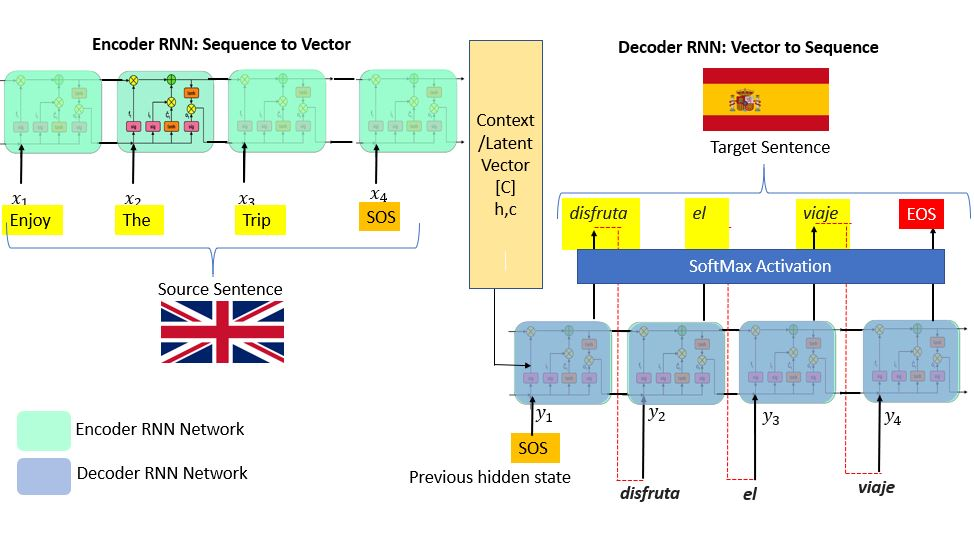This example will cover the simple implementation of seq2seq modeling in Keras. I would suggest running the model on GPU. You can take advantage of Google Colab's free GPU feature.

Go to Edit, then Notebook Settings, make changes, and save .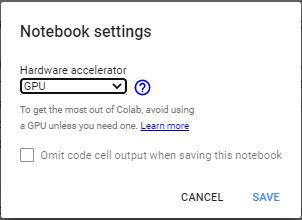``````1from google.colab import drive
2drive.mount('/content/drive')``````
python

Copy and paste the authentication code and press enter.

Set up an environment, install the libraries, and define the parameters:

``````1import tensorflow as tf
2from tensorflow import keras
3from keras.layers import *
4from keras.models import *
5from keras.utils import *
6from keras.initializers import *
7from keras.optimizers import * ``````
python

Define the parameter and set up the path for the `spa.txt` file you downloaded earlier on your drive. Define batch size, epochs to train for, LSTM latent dimensionality for the encoder, and the number of samples.

``````1batch_size = 64
2epochs = 100
3latent_dim = 256
4num_samples = 10000
5# set the data_path accordingly
6data_path = "/content/drive/My Drive/spa.txt" ``````
python

Change the `data_path` accordingly.

## Pre-processing

You won't be required to conduct in-depth text pre-processing steps. But if you want to know more about noises associated and text pre-processing, kindly refer to this Importance of Text Processing guide. You can use tokenization; its job is to convert the input sentence into a sequence of integers. To achieve this, pass your data by using Keras’s `Tokenizer()` class.

Next, vectorize the data. It will read each line and append a list to it. The top three lines are below .

``````1input_texts = []
2target_texts = []
3input_characters = set()
4target_characters = set()
5with open(data_path, "r", encoding="utf-8") as f:
python

This example sets the parameter to 10,000 samples. The first two lines of the code below will put the English text in the `input_text` and Spanish text in `target_text`.

``````1for line in lines[: min(num_samples, len(lines) - 1)]:
2    input_text, target_text, _ = line.split("\t")
3    ############### A ###############
4    target_text = "\t" + target_text + "\n"
5    input_texts.append(input_text)
6    target_texts.append(target_text)
7    ############### B ###############
8    for char in input_text:
9        if char not in input_characters:
11    for char in target_text:
12        if char not in target_characters:
14print(input_characters)
15print(target_characters)``````
python

The next step is to define the start and the end of sequence character using tab ( `\t` ) at the start of the character and `\n` at the end of the character.

Along with the English and Spanish text, you'll also want a list of their unit characters. The corresponding list output is below.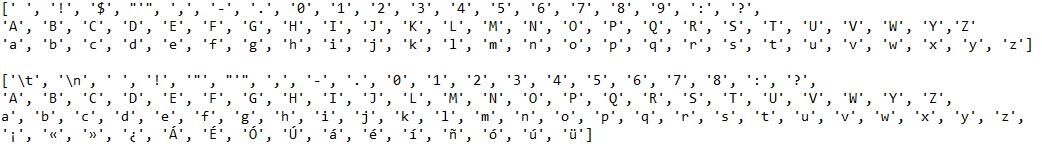Define the parameters. They are important while building the model and feature engineering.

``````1input_characters = sorted(list(input_characters))
2target_characters = sorted(list(target_characters))
3num_encoder_tokens = len(input_characters)
4num_decoder_tokens = len(target_characters)
5max_encoder_seq_length = max([len(txt) for txt in input_texts])
6max_decoder_seq_length = max([len(txt) for txt in target_texts])
7
8print("No.of samples:", len(input_texts))
9print("No.of unique input tokens:", num_encoder_tokens)
10print("No.of unique output tokens:", num_decoder_tokens)
11print("Maximum seq length for inputs:", max_encoder_seq_length)
12print("Maximum seq length for outputs:", max_decoder_seq_length)``````
python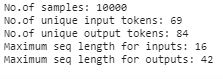Now that you have a list of the characters, perform index mapping to input and target it.

``````1input_token_index = dict([(char, i) for i, char in enumerate(input_characters)])
2target_token_index = dict([(char, i) for i, char in enumerate(target_characters)])
3
4print(input_token_index)
5print(target_token_index)``````
python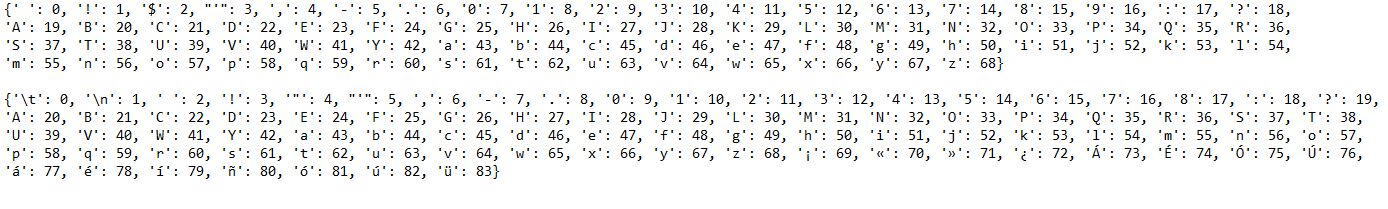Notice that each character is now associated with an integer value.

Refer the Keras documentation on pre-processing for more detail.

## Feature Engineering

To generate feature vectors, on-hot encoding is used. Turn 3D numpy arrays to store one-hot encoding. To generate the feature's variables, `encoder_input_data`, `decoder_input_data`, `decoder_target_data` are used. `encoder_input_data` and `decoder_input_data` contain one-hot vectorization of English and Spanish sentences, respectively.

The first dimension, `input_texts`, states the number of sample texts (10,000 in this case). The second dimension, `max_encoder_seq_length` (English) and `max_decoder_seq_length` (Spanish), is the longest encoder/decoder sequence length within the samples. The third dimension, `num_encoder_tokens` (English) and `num_decoder_tokens` (Spanish), contains unique characters in `input_charaters` and `output_characters`.

The `decoder_target_data` is like `decoder_input_data`, the only difference is that the `decoder_target_data` is offset by one timestamp. The `decoder_target_data[:, t, :]` is the same as `decoder_input_data[:, t + 1, :]` .

Now that everything is set, build the model and put the above variables and feature vectors to their proper encoder-decoder model.

``````1encoder_input_data = np.zeros(
2  (len(input_texts), max_encoder_seq_length, num_encoder_tokens), dtype="float32"
3)
4
5decoder_input_data = np.zeros(
6  (len(input_texts), max_decoder_seq_length, num_decoder_tokens), dtype="float32"
7)
8
9decoder_target_data = np.zeros(
10  (len(input_texts), max_decoder_seq_length, num_decoder_tokens), dtype="float32"
11)
12
13for i, (input_text, target_text) in enumerate(zip(input_texts, target_texts)):
14    for t, char in enumerate(input_text):
15        encoder_input_data[i, t, input_token_index[char]] = 1.0
16    encoder_input_data[i, t + 1 :, input_token_index[" "]] = 1.0
17    for t, char in enumerate(target_text):
18        decoder_input_data[i, t, target_token_index[char]] = 1.0
19        if t > 0:
20            decoder_target_data[i, t - 1, target_token_index[char]] = 1.0
21    decoder_input_data[i, t + 1 :, target_token_index[" "]] = 1.0
22    decoder_target_data[i, t:, target_token_index[" "]] = 1.0``````
python

## Conclusion

The fundamental idea of this guide was to give a brief understanding of the seq2seq model, encoder, and decoder. This guide will help you take this to the next level by teaching you how to build a model using LSTM RNN.

You can now choose any language of your choice. Just download the language you want to translate and define a proper path of the data. Before moving further, make sure you understand LSTM well. Feel free to ask at Codealphabet if you have any queries regarding this guide.